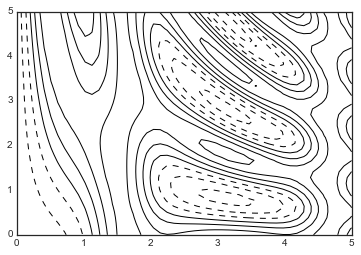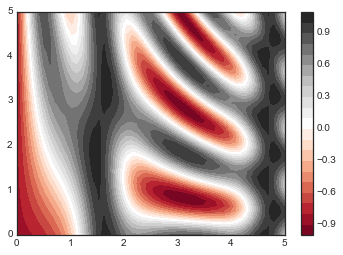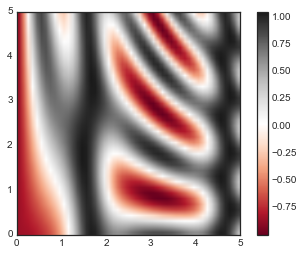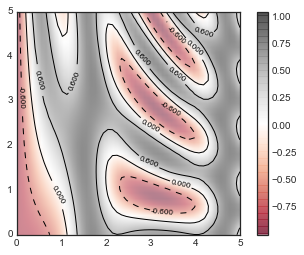# 8.7 密度和等高线图

``````%matplotlib inline
import matplotlib.pyplot as plt
plt.style.use('seaborn-white')
import numpy as np
``````

## 可视化三维函数

``````def f(x, y):
return np.sin(x) ** 10 + np.cos(10 + y * x) * np.cos(x)
``````

``````x = np.linspace(0, 5, 50)
y = np.linspace(0, 5, 40)

X, Y = np.meshgrid(x, y)
Z = f(X, Y)
``````

``````plt.contour(X, Y, Z, colors='black');
````````````plt.contour(X, Y, Z, 20, cmap='RdGy');
````````````plt.cm.<TAB>
``````

``````plt.contourf(X, Y, Z, 20, cmap='RdGy')
plt.colorbar();
````````````plt.imshow(Z, extent=[0, 5, 0, 5], origin='lower',
cmap='RdGy')
plt.colorbar()
plt.axis(aspect='image');
``````• `plt.imshow()`不接受`x``y`网格，所以你必须在绘图上手动指定图像的边界`[xmin, xmax, ymin, ymax]`
• `plt.imshow()`默认遵循标准图像数组定义，其中原点位于左上角，而不是大多数等高线图中的左下角。 显示网格化数据时必须更改此值。
• `plt.imshow()`将自动调整轴纵横比来匹配输入数据；这可以通过设置，例如`plt.axis（aspect ='image'）`来使`x``y`单位匹配来更改。

``````contours = plt.contour(X, Y, Z, 3, colors='black')
plt.clabel(contours, inline=True, fontsize=8)

plt.imshow(Z, extent=[0, 5, 0, 5], origin='lower',
cmap='RdGy', alpha=0.5)
plt.colorbar();
``````